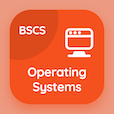Business Statistics Quiz PDF - Complete

# Discrete Probability Distributions Multiple Choice Questions p. 14

Study Discrete Probability Distributions multiple choice questions and answers, discrete probability distributions quiz answers PDF 14 to study Business Statistics course online. Probability Distributions MCQ trivia questions, discrete probability distributions Multiple Choice Questions (MCQ) for online college degrees. "Discrete Probability Distributions MCQ" PDF eBook: probability rules, principles of measurement, discrete probability distributions test prep for online BBA degree.

"The tail or head, the one or zero and the girl and boy are examples of" MCQ PDF: complementary events, non-functional events, non complementary events, and functional events for bachelor's degree in business. Learn probability distributions questions and answers to improve problem solving skills for business management degree online.

## Discrete Probability Distributions Questions and Answers MCQs

MCQ: The tail or head, the one or zero and the girl and boy are examples of

non-functional events
complementary events
non complementary events
functional events

MCQ: The scale which categorize the events in collectively exhaustive manner and mutually exclusive manner is classified as

discrete scale
continuous scale
valid scale
nominal scale

MCQ: Consider two events X and Y, the X-bar and Y-bar represents

occurrence of Y
occurrence of X
non-occurrence of X and Y
occurrence of X and Y

MCQ: The value of Σfx is 300, A= 35, number of observations are 15 and width of class interval is 5 then arithmetic mean is

135
145
150
235

MCQ: In measuring the probability of any certain event, the zero represents

impossible events
possible events
certain event
sample event

### More Quizzes from Business Statistics Course